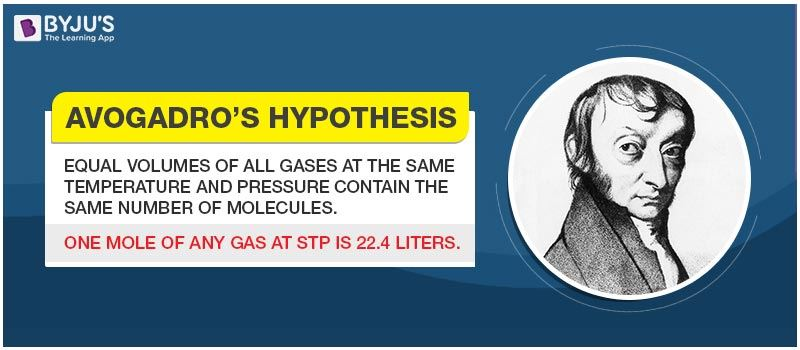# Avogadro's Hypothesis

Avogadro built on the work of Gay-Lussac who first noted that gases reacted in simple integer volume ratios (when measured at the same temperature and pressure). Gay-Lussac concluded that this result could be explained if the volumes contained the same number of particles. Chemists found this hypothesis difficult to accept because they reasoned, for example, that if the particles of the gases were combining, then one volume of gas A reacting with one volume of gas B should produce one volume of product. However, it was frequently found that one volume of gas A reacted with one volume of gas B to produce two volumes of product. Chemists, including Gay-Lussac, were unable to account for this behavior of gases.

Avogadro reasoned that these results could be explained if Gay-Lussac’s particles were actually combinations of smaller particles (which we now call “atoms”). He introduced the use of the term “molecule” to refer to these combinations of smaller particles. “Molecule” comes from “mole” lumps of matter and “cula” little. A molecule is a little lump of matter. His hypothesis was consistent with the view that gas pressure is caused by the collisions of molecules with the side of the container. If the pressure is the same, then it seems reasonable that the number of molecules in the container is the same. In addition to the ammonia and hydrogen chloride example combining in a one to one ratio, he found that a number of gases combined in such a way that could only be accounted for if the individual molecules were diatomic.

The diagram below shows how one volume of hydrogen reacts with one volume of chlorine to produce two volumes of hydrogen chloride. Making the assumption that the gases are diatomic neatly accounts for the experimental evidence.Of course, Avogadro did not base his hypotheses about number of molecules in a volume and the diatomic nature of molecules on just a couple examples. He cited the results of many experiments. Still, the scientific community was not able to accept the validity of his hypothesis until many years after his death. Using his hypothesis, chemists were able to deduce the formulas of gaseous substances based on combining volumes. The use of this hypothesis was also instrumental in determining the molar mass of elements.

### Avogadro’s Law:

In 1811 an Italian chemist Amedeo Avogadro stated that “ equal volumes of gases at the same temperature and pressure contain the same number of molecules regardless of their chemical nature and physical properties”. This number is called Avogadro’s number.

Mathematically it can be represented as follows,

$\frac{V}{n}=k$

Where,

V = the volume of the gas

n = the amount of substance of the gas

k = proportionality constant

### Avogadro’s Number:

It is the number of molecules of any gas present in a volume of 22.41 L and it is same for the lightest gas i.e. hydrogen as for a heavy gas such as carbon dioxide or bromine. Its value is $6.022\times 10^{23}$.

 Read More About Avagadro’s Number Avagadro’s Number

Stay tuned with Byju’s to learn more about Avogadro’s hypothesis, Avogadro’s law and much more.